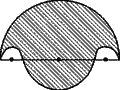#The boundary of the shaded region in the figure given  consists of four semi-circular arcs, the smallest two being equal. If the diameter of the larger is 14 cm and of the smallest is 3·5 cm, calculate the length of the boundary and the area of the shaded region. (Take= 22/7).In the given figure, PS = 14 cm is a diameter of a circle and PQ = 3.5 cm

The length of PQ and RS are equal,

So,And radius of the semicircle PTSP,The length of the boundary = 2×Perimeter of the semicircle PBQPPerimeter of the semicircle PTSPPerimeter of the semicircle QERQHence the length of the boundary will be 44 cm.

Now,

Area of the shaded region = Area of the semicircle PTSP2×Area of the semicircle PBQP+ Area of the semicircle QESQHence area of the shaded region will be 86.546 cm2.

• 9

length of boundary:

perimeter of (biggest semicircle + middle + 2* smallest semicircle)= length of boundary

length of boundary = pi (7 + 3.5 + 3.5)

length of boundary = 22/7*14

length of boundary = 44cm2

• -9

length of boundary:

length of boundary = area of (biggest semicircle + middle circle - 2*smallest circle)

length of boundary =  pi(72+ 3.5-3.5)

length of boundary = 22/7*49

length of boundary = 154 cm 2

• -15

srry its 44 cm,

• 1

ooops!

u got it rong

• -16

pls check and post correct answer...

• -12

what wrong...???

• -10

length of boundary:

perimeter of (biggest semicircle + middle + 2* smallest semicircle)= length of boundary

2pi r1 + 2pi r2 + 2 pi r3 = pi ( d1 + d2 + d3)

length of boundary = pi (7 + 3.5 + 3.5)

length of boundary = 22/7*14

length of boundary = 44cm2

• 14

sorry i dont need perimeter just area of the shaded region  :)

• -16

ya ya boundary is right

how to find area of shaded region???????

• -21

• -16

(area of biggest (radius = 7cm)semicirclie - area of two smallest (radius= 3.5/2) semicircles) + area of small (of radius 3.5 cm) semi-circle

= calculate

• 2

i did it but not gettin right aner

correct area is 72.2 cm2

• -19

how can the length b in cm2

• -7
What are you looking for?